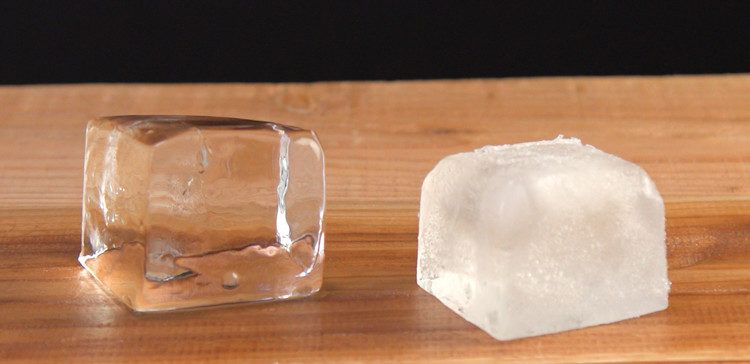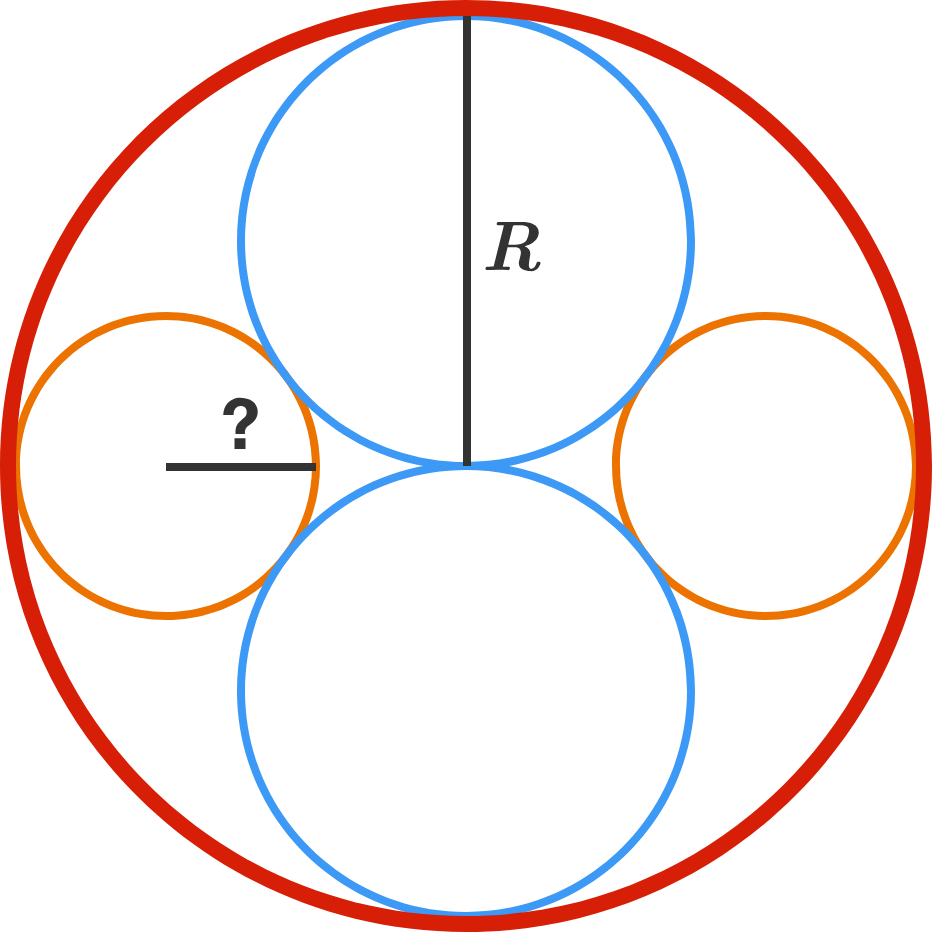# Problems of the Week

Contribute a problem

# 2018-02-19 BasicThe animation shows a football player, Josh Imatorbhebhe, jumping high in the air.

He swings his arms in the air and appears to levitate in the air for a brief moment of time.

How does swinging his arms help him accomplish this feat?

Once I went to a fancy restaurant where the ice cubes were clear like a glass of water. When I make them at home, they're opaque and I can't see through them.The iced drinks I make are never as pretty as the ones at the restaurant. Which of the following approaches might make my ice cubes more clear?

We know that $\underbrace{4 \times 4 \times 4 \times 4}_{4 \text{ times}} = 4^4.$

But how many $4^4$ are there in the left side of the equality $\underbrace{4^4 \times 4^4 \times \cdots \times 4^4}_{\text{How many times?}} = {\large 4^{\left( 4^4 \right)}} ?$There are two identical blue circles and two identical orange circles arranged symmetrically in a larger red circle, as shown. The circles are tangent to each other where they touch.

If the radius of the red circle is $R,$ then what is the radius of one of the orange circles?

Hint: Try finding a right triangle and applying the Pythagorean theorem.

In the $MIU$ system, you always start with the string $MI$ and then form new strings by applying any of following rules any number of times:

 Rules Examples 1. If your string ends with $I$, you can add a $U$ at the end. $MI\longrightarrow MIU$ 2. You can double the entire string following $M.$ $MIU\longrightarrow MIUIU$ 3. Three consecutive $I$'s can be replaced with a single $U.$ $MIIIU\longrightarrow MUU$ 4. Two consecutive $U$'s can be removed from the string. $MUU\longrightarrow M$

Which of the following strings can be derived from $MI$ using these rules?

Note: None of the rules can be used in the opposite way; for example, you aren't allowed to derive $MUUI$ from $MI$ by using a reversed version of rule 4.

Source: The $MIU$ puzzle was introduced by Douglas Hofstadter in his magisterial work Gödel, Escher, Bach.

×